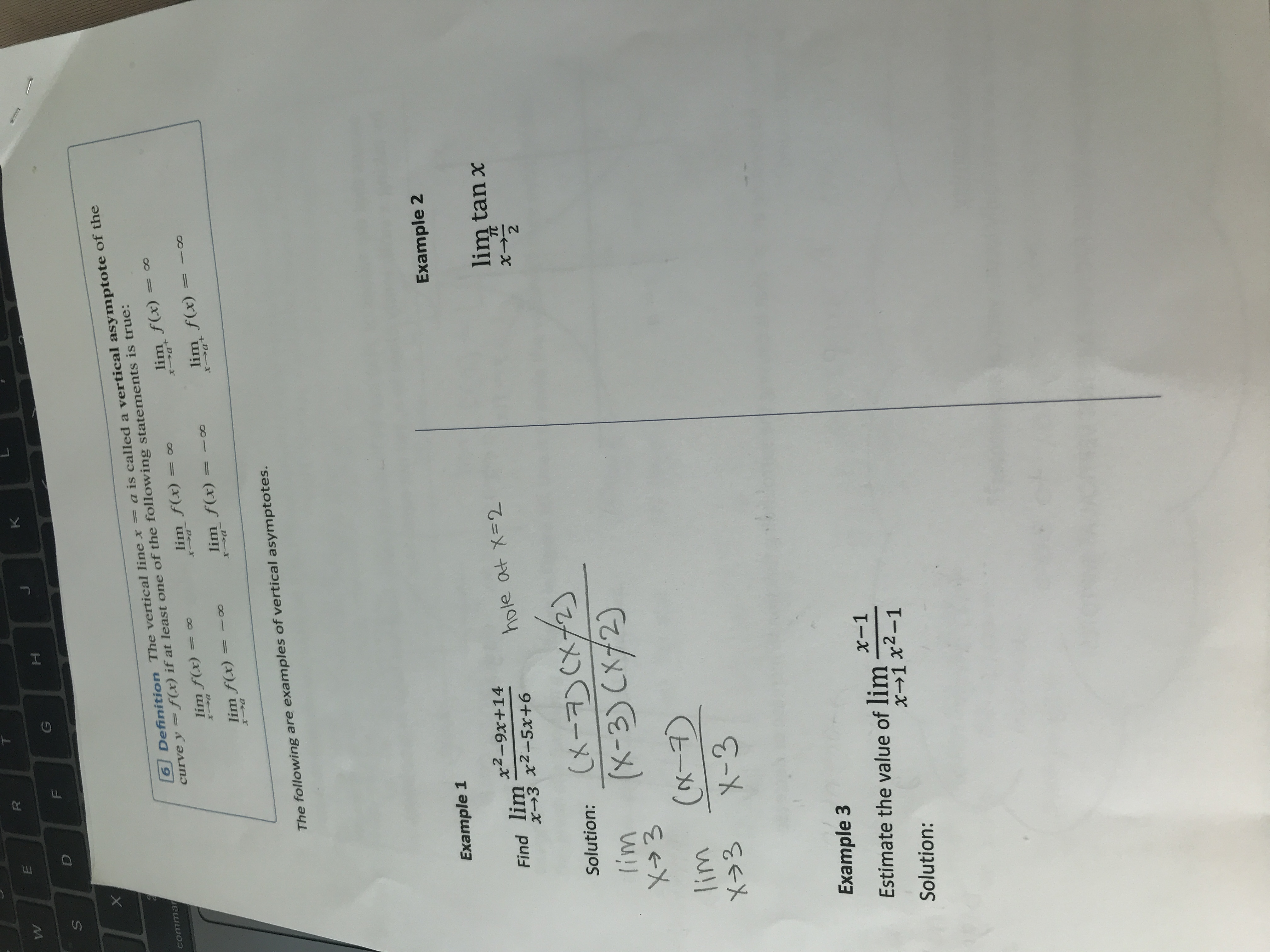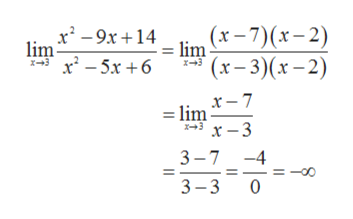RWKGDa is called a vertical asymptote of thecommarcurve y = f(x) if at 1least one of the following statements is true:lim f(x)6 Definition The vertical line x00lim f(x)lim f(x)XJa+De-rXJClim f(x)x atlim f(x)lim f(x)The following are examples of vertical asymptotes.Example 1Example 2Find lim2-9x+ 14X3hole at X-2lim tan xTC22Solution: X7CX21IimX3(X-3) C2)lim Cx-X-3X3Example 3Estimate the value of lim2-1x-1x-1 xSolution:U.X

Question

Please answer the examples and show your work. Thank you!help_outlineImage TranscriptioncloseR W K G D a is called a vertical asymptote of the commar curve y = f(x) if at 1least one of the following statements is true: lim f(x) 6 Definition The vertical line x 00 lim f(x) lim f(x) XJa +De-r XJC lim f(x) x at lim f(x) lim f(x) The following are examples of vertical asymptotes. Example 1 Example 2 Find lim2-9x+ 14 X3 hole at X-2 lim tan x TC 22 Solution: X7CX21 Iim X3 (X-3) C2) lim Cx- X-3 X3 Example 3 Estimate the value of lim2-1 x-1 x-1 x Solution: U. X fullscreen
Step 1

1). Given,

Step 2

On simplification, we gethelp_outlineImage Transcriptioncloselim (t-7)(r-2) '(x-3)(x-2) x29x14 lim X-3 x2-5x 6 x3 x- 7 -lim x -3 x+3 3-7 -4 3-3 0 Tlo II fullscreen
Step 3

2). Given,

&nb...

Want to see the full answer?

See Solution

Want to see this answer and more?

Our solutions are written by experts, many with advanced degrees, and available 24/7

See Solution
Tagged in

Calculus